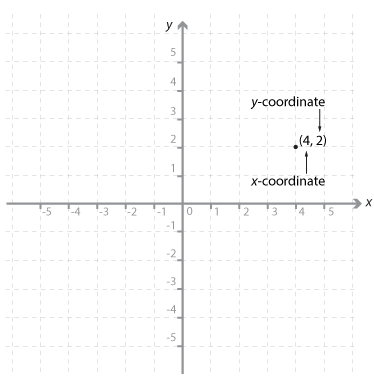# Year 9

## Number and Algebra

### Linear and non-linear relationships

Coordinate geometry has traditionally been attributed to René Descartes (1599−1650) and Pierre de Fermat (1601−1665) who independently provided the beginning of the subject as we know it today. It is one of the most important and exciting ideas of mathematics. In particular, it is central to the mathematics students meet at school. It provides a connection between algebra and geometry through graphs of lines and curves. This enables geometric problems to be solved algebraically and provides geometric insights into algebra.

### Cartesian plane

The number plane (Cartesian plane) is divided into four quadrants by two perpendicular axes called the x-axis (horizontal line) and the y-axis (vertical line). These axes intersect at a point called the origin. The position of any point in the plane can be represented by an ordered pair of numbers (x, y). These ordered pairs are called the coordinates of the point.Detailed description

The point with coordinates (4, 2) has been plotted on the Cartesian plane shown. The coordinates of the origin are (0, 0).
Once the coordinates of two points are known, the distance between the two points and the midpoint of the interval joining the points can be found.